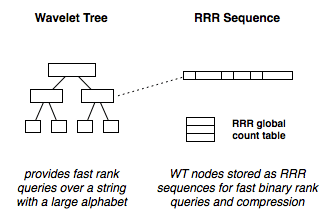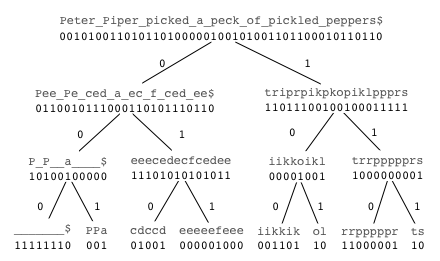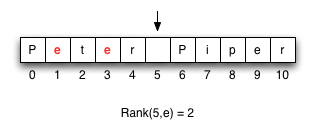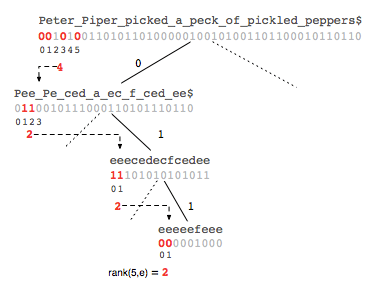# Wavelet Trees: an IntroductionToday I will talk about an elegant way of answering rank queries on sequences over larger alphabets – a structure called the Wavelet Tree. In my last post I introduced a data structure called RRR, which is used to quickly answer rank queries on binary sequences, and provide implicit compression.

A Wavelet Tree organises a string into a hierarchy of bit vectors. A rank query has time complexity is $\mathcal{O}(\log_2{A})$, where $A$ is the size of the alphabet. It was introduced by Grossi, Gupta and Vitter in their 2003 paper High-order entropy-compressed text indexes  (see the Further Reading section for more papers). It has since been featured in many papers [1, 2, 3, 5, 6].

If you store the bit vectors in RRR sequences, it may take less space than the original sequence. Alternatively, you could store the bit vectors in the rank indexes proposed by Sadakane and Okonohara . It has a different approach to compression. I will talk about it another time ;) – fortunately, I will be studying under Sadakane-sensei at a later date (update: now I’m doing my Ph.D. under him in Tokyo).

In a different future post, I will show how Suffix Arrays can be used to find arbitrary patterns of length $P$, by issuing $2P$ rank queries. If using a Wavelet Tree, this means a pattern search has $\mathcal{O}(P \log_2{A})$ time complexity, that is, the size of size of the ‘haystack’ doesn’t matter, it instead depends on the size of the ‘needle’ and size of the alphabet.

## Constructing a Wavelet Tree

A Wavelet Tree converts a string into a balanced binary-tree of bit vectors, where a $0$ replaces half of the symbols, and a $1$ replaces the other half. This creates ambiguity, but at each level this alphabet is filtered and re-encoded, so the ambiguity lessens, until there is no ambiguity at all.

The tree is defined recursively as follows:

1. Take the alphabet of the string, and encode the first half as $0$, the second half as $1$: $\{ a, b, c, d \}$ would become $\{ 0, 0, 1, 1 \}$;
2. Group each $0$-encoded symbol, $\{ a, b \}$, as a sub-tree;
3. Group each $1$-encoded symbol, $\{ c, d \}$, as a sub-tree;
4. Reapply this to each subtree recursively until there is only one or two symbols left (when a $0$ or $1$ can only mean one thing).

For the string "Peter Piper picked a peck of pickled peppers" (spaces and a string terminator have been represented as $\_$ and $\$$respectively, due to convention in the literature) the Wavelet Tree would look like this:note: the strings aren’t actually stored, but are shown here for convenience It has the alphabet \{ \, P, \_, a, c, d, e, f, i, k, l, o, p, r, s, t \}, which would be mapped to \{ 0, 0, 0, 0, 0, 0, 0, 0, 1, 1, 1, 1, 1, 1, 1, 1 \}. So, for example, \$$ would map to$0$, and$r$would map to$1$. The left subtree is created by taking just the 0-encoded symbols$\{ \$, P, \_, a, c, d, e, f \}$ and then re-encoding them by dividing this new alphabet: $\{ 0, 0, 0, 0, 1, 1, 1, 1 \}$. Note that on the first level an $e$ would be encoded as a $0$, but now it is encoded as a $1$ (it becomes a $0$ again at a leaf node).

We can store the bit vectors in RRR structures for fast binary rank queries (which are needed, as described below), and compression :) In fact, since it is a balanced tree, we can concatenate each of the levels and store it as one single bit vector.

## Querying a Wavelet Tree

Recall from my last post that a rank query is the count of $1$-bits up to a specified position. Rank queries over larger alphabets are analogous – instead of a $1$, it may be any other symbol:After the tree is constructed, a rank query can be done with log $A$ ($A$ is alphabet size) binary rank queries on the bit vectors – $\mathcal{O}(1)$ if you store them in RRR or another binary rank index. The encoding at each internal node may be ambiguous, but of course it isn’t useless – we use the ambiguous encoding to guide us to the appropriate sub-tree, and keep doing so until we have our answer.

For example, if we wanted to know $rank(5, e)$, we use the following procedure which is illustrated below. We know that $e$ is encoded as $0$ at this level, so we take the binary rank query of $0$ at position $5$:Which is $4$, which we then use to indicate where to rank in the $0$-child: the $4^{th}$ bit (or the bit at position $3$, due to $0$-basing). We know to query the $0$-child, since that is what $e$ was encoded as at the parent level. We then repeat this recursively:At a leaf node we have our answer. I would love to explain why this works, but it is fun and rewarding to think about it yourself ;)

There are also ways to provide fast select queries, but once again I will leave that up to you to research. The curious among you might also be interested in the Huffman-Shaped Wavelet Tree described by Mäkinen and Navarro .

## Using Your New Powers for Good

Feel free to implement this yourself, but if you want to get your hands dirty right away, all-around-clever-guy Francisco Claude has made an implementation available in his Compressed Data Structure Library (libcds). If you create something neat with it be sure to report back ;)

Update: Terence Siganakis wrote a blog post about Wavelet Trees that made it to the front page of Hacker News, encouraging an interesting discussion. The discussion is here.

And if you read this far, consider following me on Twitter: @alexbowe.

I didn’t want to saturate this blog post with proofs and other details, since it was meant to be a light introduction. If you want to dive deeper into this beautiful structure, check out the following papers:

 F. Claude and G. Navarro. Practical rank/select queries over arbitrary sequences. In Proceedings of the 15th International Symposium on String Processing and Information Retrieval (SPIRE), LNCS 5280, pages 176–187. Springer, 2008.

 P. Ferragina, R. Giancarlo, and G. Manzini. The myriad virtues of wavelet trees. Information and Computation, 207(8):849–866, 2009.

 P. Ferragina, G. Manzini, V. M ̈akinen, and G. Navarro. Compressed representations of sequences and full-text indexes. ACM Transactions on Algorithms, 3(2):20, 2007.

 R. Grossi, A. Gupta, and J. Vitter. High-order entropy-compressed text indexes. In Proceedings of the 14th annual ACM-SIAM symposium on Dis- crete algorithms, pages 841–850. Society for Industrial and Applied Mathematics, 2003.

 V. Mäkinen and G. Navarro. Succinct suffix arrays based on run-length encoding. Nordic Journal of Computing, 12(1):40–66, 2005.

 V. Mäkinen and G. Navarro. Implicit compression boosting with applications to self-indexing. In Proceedings of the 14th International Symposium on String Processing and Information Retrieval (SPIRE), LNCS 4726, pages 214–226. Springer, 2007.

 D. Okanohara and K. Sadakane. Practical entropy-compressed rank/select dictionary. Arxiv Computing Research Repository, abs/cs/0610001, 2006.

data structures

### Alex Bowe Twitter

Alex has a PhD in succinct data structures for bioinformatics, and currently works on self-driving cars. He's also interested in cryptocurrency, business, fashion, photography, teaching, and writing.

## Succinct de Bruijn Graphs

This post will give a brief explanation of a Succinct implementation for storing de Bruijn graphs [http://en.wikipedia.org/wiki/De_Bruijn_graph], which is recent (and continuing) work I have been doing with Sadakane. Using our new structure, we have squeezed a graph for a human genome (which

## FM-Indexes and Backwards Search

Last time (way back in June! I have got to start blogging consistently again) I discussed a gorgeous data structure called the Wavelet Tree [https://alexbowe.com/wavelet-trees/]. When a Wavelet Tree is stored using RRR sequences, it can answer rank and select operations in $\mathcal{O}(\log{A})$ time,

## RRR: A Succinct Rank/Select Index for Bit Vectors

This blog post will give an overview of a static bitsequence data structure known as RRR, which answers arbitrary length rank queries in $\mathcal{O}(1)$ time, and provides implicit compression. As my blog is informal, I give an introduction to this structure from a birds eye view. If you